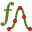# Analytical Curve

Create an analytical curve to be used either as a building block for constructing geometry or as a wire. Analytical curves are parametric definitions (in the parameter “t”) that define the path in three coordinate systems.

The derivatives of the expressions are required and need to exist over the entire path. If dividing the derivative by zero, the definition is not accepted. An alternative would be to calculate the points in the scripting environment and create a fitted spline.

On the Construct tab, in the Create Curve group, click theAnalytical Curve icon.
Tip: Press V,5 to use the shortcut key.Parametric interval Interval over which the analytical curve is parametrically defined. Cartesian description The description of the curve using the Cartesian coordinate system. The U, V and N dimensions as a function of variable t.Parametric interval Interval over which the analytical curve is parametrically defined. Cylindrical description The cylindrical description of the curve in the ρ, θ and ϕ dimensions as a function of variable t.Parametric interval Interval over which the analytical curve is parametrically defined. Spherical description The spherical description of the curve in the r, θ and ϕ dimensions as a function of variable t.